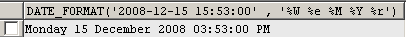Tutorials

# Sql Date and Time Format

The Tutorial illustrate an example from the 'Sql Date and Time Format'. To understand this example we use the following syntax that is used to format date and time.

The Tutorial illustrate an example from the 'Sql Date and Time Format'. To understand this example we use the following syntax that is used to format date and time.

# Sql Date and Time Format

The Tutorial illustrate an example from the 'Sql Date and Time Format'. To understand this example we use the following syntax that  is used to  format date and time.

SYNTAX:- DATE_FORMAT(date,format)

date:-This is the field in  which we have specified date and time.

format:-The format specifier used here are

%W:-   This is used for showing weekday name i.e(Sunday-Saturday).
%e:-   This is used for showing Day of the month, numeric (0-31).
%M :-  This is used for showing Month name (January-December)
%Y:-  This is used for showing Year, numeric, four digits
%r :-  This is used for showing Time, 12-hour (hh:mm:ss followed by AM or PM)

Query:-

The given below Date_Format(date,format) Query return you the format date in the order of Week,Day,Month,Year and time.

 SELECT DATE_FORMAT('2008-12-15 15:53:00','%W %e %M %Y %r');

Output:-